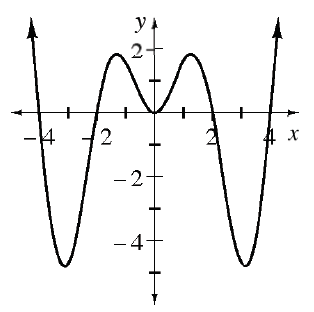### Home > APCALC > Chapter 4 > Lesson 4.1.1 > Problem4-7

4-7.

Examine the function, $f$, graphed at right.

1. Is $f$ even, odd, or neither?

Even functions have reflective symmetry across the $y$-axis. Odd functions have rotational symmetry about the origin.

2. If $\int _ { 0 } ^ { 2 } f ( x ) d x = 10$, what is $\int _ { - 2 } ^ { 2 } f ( x ) d x$? Explain.

$\int_{0}^{2}f(x)dx = 10 \text{ means the exact area under }f(x)\text{ between }x=-2 \text{ and }x=2\text{ is }10.$

$\text{Since }f(x)\text{ is even, }\int_{0}^{2}f(x)dx=\int_{-2}^{0}f(x)dx=10.$

$\text{and }f(x)\int_{-2}^{2}f(x)dx=2\int_{0}^{2}f(x)dx=20.$

3. If $\int _ { 0 } ^ { 3 } f ( x ) d x = - 2$ and $\int _ { 0 } ^ { 2 } f ( x ) d x = 10$, what is the value of $\int _ { 2 } ^ { 3 } f ( x ) d x$? Explain.

$\int_{2}^{3}f(x)dx=\int_{0}^{3}f(x)dx-\int_{0}^{2}f(x)dx=$

4. If you know the values of $\int _ { 0 } ^ { 3 } f ( x ) d x$ and $\int _ { - 2 } ^ { 2 } f ( x ) d x$, how can you determine the value of $\int _ { 2 } ^ { 3 } f ( x ) d x$? Justify your process with a diagram, if necessary.

$\text{If you know}\int_{-2}^{2}f(x)dx\text{ then you know }\int_{0}^{2}f(x)dx$

Refer to the steps in part (c) for more guidance.